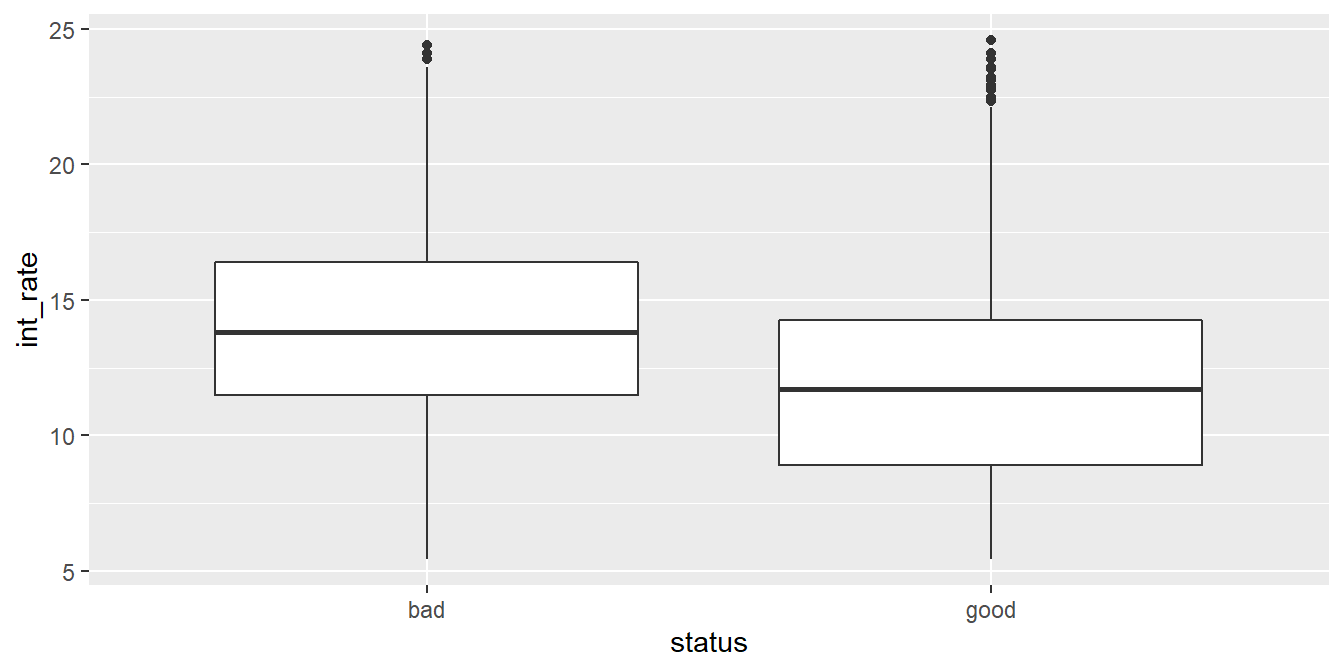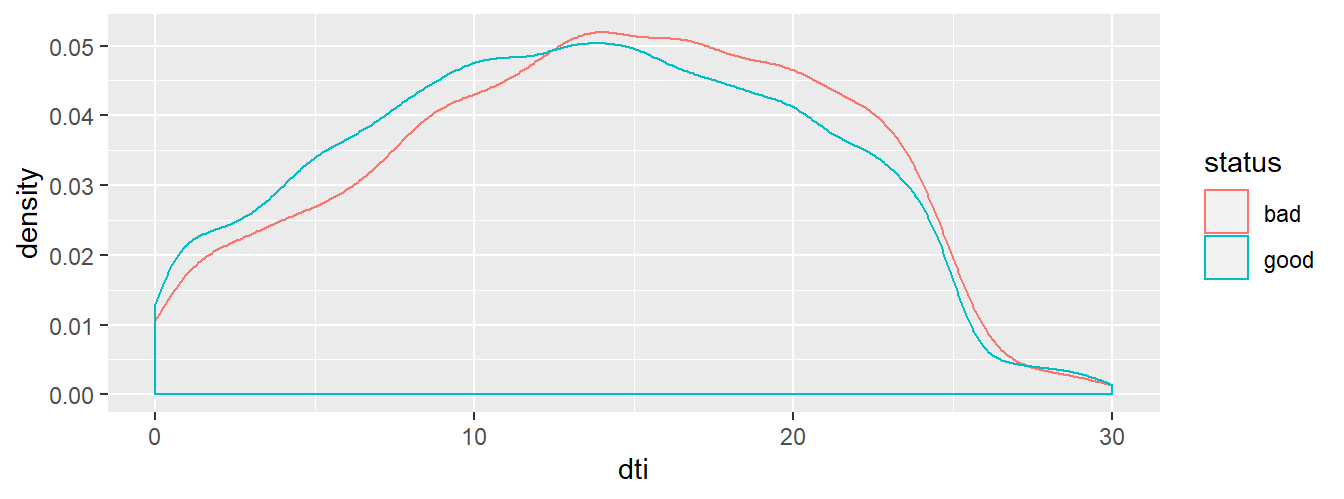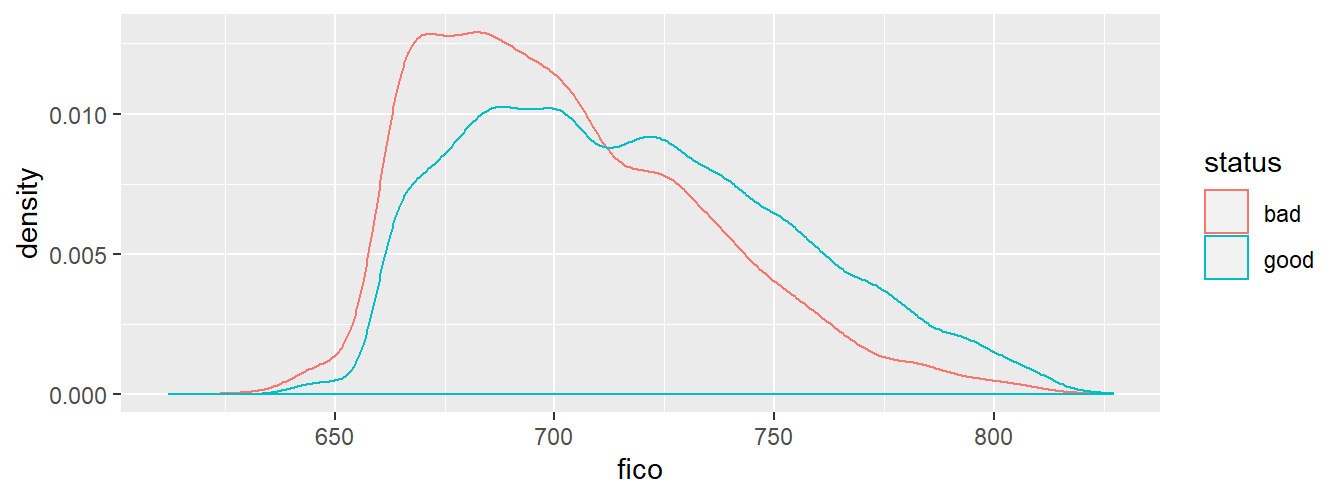# Lab 8: Application of Nearest Neighbor Algorithm

### Learning objectives

• cleaning/exploring data
• replacing characters in a character variables using gsub()
• finding predictors/features
• normalizing quantitative variables
• creating a function in R usiong function(x)
• creating train and test data sets using sample()
• making and evaluating k-NN predictions

### 1. Cleaning/exploring data

In this lab we will attempt to predict which loans will be repaid and which will default. Assessing creditworthiness of borrowers is a classic application of machine learning: banks have plenty of data to train their models. We will work with data on loans from a peer-to-peer lending website Lending Club. Lending Club is one of many peer-to-peer lending platforms. Others include Prosper and Upstart. These sites deploy their own algorithms to accept or reject the loan, and to set the interest rate. Our goal is to use examine the loans offered on the platform and predict which ones will default. There exists an entire industry helping investors choose loans from peer-to-peer lending platforms. For example, Lending Robot and PeerCube run and sell algorithms to select the best loans. We will try to do the same in this lab.

The data is publicly available on Lending Club’s website. (Though you may have to be a member to get the full data set.) We will work with data on loans issued between 2007 and 2011. Since the longest loan term is 5 years, most of the loans should be either paid off or delinquent. The first row in the .csv file contains a statement from Lending Club so we skip the first row by adding skip=1 as an option in the read_csv() function.

library(tidyverse)
library(stargazer)
library(descr)
loan <- read_csv("LoanStats3a_securev1.csv", skip=1)

We see that this is a rather rich data with over 40 thousand loans and 56 variables. The description of these variables is here.

The variable we would like to predict is loan_status. Let’s see what values it takes.

table(loan$loan_status) ## ## Charged Off ## 5310 ## Current ## 4012 ## Default ## 10 ## Does not meet the credit policy. Status:Charged Off ## 755 ## Does not meet the credit policy. Status:Current ## 74 ## Does not meet the credit policy. Status:Fully Paid ## 1913 ## Does not meet the credit policy. Status:In Grace Period ## 1 ## Does not meet the credit policy. Status:Late (16-30 days) ## 1 ## Does not meet the credit policy. Status:Late (31-120 days) ## 5 ## Fully Paid ## 30239 ## In Grace Period ## 64 ## Late (16-30 days) ## 12 ## Late (31-120 days) ## 139 Clearly, it is not as simple as good loan versus bad loan. Also, there are three loans with a blank “” loan status. Looking at these observations we see that many variables are missing for these three loans. Therefore, we will filter these loans out. loan <- filter(loan, loan_status!="") For our purposes we will consider “good” loans those with “Fully Paid”, “Current” and “Does not meet.. Fully Paid” status. Loans with any other status will be considered “bad”. loan$status <- ifelse(loan$loan_status == "Current" | loan$loan_status == "Fully Paid" |
loan$loan_status == "Does not meet the credit policy. Status:Fully Paid", "good","bad") table(loan$status)
##
##   bad  good
##  6371 36164

This gives us about 36 thousand good and 6 thousand bad loans.

Also, it appears that int_rate is a character variable. This is because the observations have the % sign which leads the computer to interpret the variable as a character. We can do this by removing the % sign from the using the gsub() function, and changing the type using the as.numeric() function.

loan$int_rate <- as.numeric(gsub("%","", loan$int_rate))

Let’s check if the interest rate takes reasonable values. Box plot is a popular data exploration plot because it lets us see how a numerical variable is distributed and if it has any outliers.

ggplot(loan, aes(x=status,y=int_rate)) + geom_boxplot()The upper and lower show us the 75th and 25th percentiles. The “whisker” lines extend to the lowest/highest value that is within the 1st/3rd quartile plus 1.5 times the interquartile range. Data points that are outside of that range are plotted individually. We see that most of the loans have interest rate around 9 to 16 percent. Good loans tend to have lower interest than bad loans indicating that higher interest loans are more likely to default.

IN-CLASS EXERCISE: Let’s use the box plot to examine the annual_inc variable. Are there a lot of outliers?

Let’s explore at least one qualitative variable: home_ownership.

crosstab(loan$home_ownership, loan$status, prop.r = TRUE, plot = FALSE)
##    Cell Contents
## |-------------------------|
## |                   Count |
## |             Row Percent |
## |-------------------------|
##
## ============================================
##                        loan$status ## loan$home_ownership      bad    good   Total
## --------------------------------------------
## MORTGAGE               2693   16266   18959
##                        14.2%   85.8%   44.6%
## --------------------------------------------
## NONE                      1       7       8
##                        12.5%   87.5%    0.0%
## --------------------------------------------
## OTHER                    28     108     136
##                        20.6%   79.4%    0.3%
## --------------------------------------------
## OWN                     488    2763    3251
##                        15.0%   85.0%    7.6%
## --------------------------------------------
## RENT                   3161   17020   20181
##                        15.7%   84.3%   47.4%
## --------------------------------------------
## Total                  6371   36164   42535
## ============================================

The share of good loans does not seem to vary a lot by type of home ownership.

### 2. Selecting predictor variables (aka features)

Let’s take a look two candidate variables that may predict good loans: debt to income ratio and FICO scores. For FICO scores let’s use the average of the high and low.

loan$fico <- (loan$fico_range_high+loan$fico_range_low)/2 The descriptive statistics of the two quantitative variables are below. (Notice that below filter() function is nested inside select() which is nested inside stargazer().) loan <- as.data.frame(loan) stargazer(select(filter(loan, status == "good"),dti, fico), median = TRUE, type = "text") ## ## ======================================================================= ## Statistic N Mean St. Dev. Min Pctl(25) Median Pctl(75) Max ## ----------------------------------------------------------------------- ## dti 36,164 13.259 6.733 0.000 8.050 13.310 18.570 29.990 ## fico 36,164 717.247 36.425 612 687 712 742 827 ## ----------------------------------------------------------------------- stargazer(select(filter(loan, status == "bad"),dti, fico), median = TRUE, type = "text") ## ## ================================================================= ## Statistic N Mean St. Dev. Min Pctl(25) Median Pctl(75) Max ## ----------------------------------------------------------------- ## dti 6,371 14.018 6.653 0 9.1 14.3 19.3 30 ## fico 6,371 702.599 32.094 617 677 697 722 822 ## ----------------------------------------------------------------- We see straight away that debt to income ratios are lower for good loans and the opposite is true for FICO scores. This is to be expected. Let’s also plot the densities of these two variables for good and bad loans. When plotting densities the key aesthetic is the x variable (the variable whose density we want to plot). By adding aesthetic color= ggplot will plot observations belonging to different values of the variable specified in color in different colors. ggplot(aes(x = dti, color = status) ,data = loan) + geom_density()ggplot(aes(x = fico, color = status) ,data = loan) + geom_density()The graphs confirm that debt-to-income ratios tend to be higher for bad loans, and FICO scores are lower for bad loans. With FICO scores we also see a sharp drop off around FICO score of 650 suggesting that Lending Club does not approve loans from borrowers with FICO below 650. ### 3. Normalize quantitative variables Since the k-NN algorithm uses Euclidean distance, it is sensitive to the scale of different variables. A variable that is measured in millions has much higher influence on the overall Euclidean distance than a variable measured in tens. Therefore, it is typical to normalize or re-scale all variables so that their magnitudes are comparable. In order for us not to have to retype a long formula several times, we will write our own function and then apply it to our predictors. normalize <- function(x) { return ((x - min(x)) / (max(x) - min(x))) } This bit of code defines a new function which we called normalize(). This function takes a vector and returns a vector whose elements have been transformed according to the formula inside the function. The formula ensures that the new vector has elements between zero and one. Let’s apply this to our quantitative variables: loan <- loan %>% mutate(fico_n = normalize(fico), dti_n = normalize(dti)) summary(select(loan, fico, fico_n)) ## fico fico_n ## Min. :612.0 Min. :0.0000 ## 1st Qu.:687.0 1st Qu.:0.3488 ## Median :712.0 Median :0.4651 ## Mean :715.1 Mean :0.4793 ## 3rd Qu.:742.0 3rd Qu.:0.6047 ## Max. :827.0 Max. :1.0000 ### 4. Split loan data into test and train We will use 80% of the loan data to train our model and the rest we will use to test our predictions. Since selecting the test and train observations is random, we will ‘set seed’ so that the computer generates the same set of random numbers each time we run this program. This makes our results reproducible. We will use function sample(x,n) to create a column of n random numbers between 1 and x. Our x is equal to the number of observations in loan. Our n is 80% of the number of observations in loan - if loan has 42,535 observations, we want 42,535*0.8=34,028 random numbers. sample(42535,34028) will generate a column of 34,028 random numbers that range between 1 and 42,535. We want the code to work for any data set so instead of hard-coding the numbers we use nrow(loan) to get 42,535, and floor(nrow(loan)*0.8) to get 34,028. We use the floor() function to round down in case nrow(loan)*0.8 is not an integer. floor(x) returns the the whole number part of x, e.g. floor(3.6)=3. set.seed(364) sample <- sample(nrow(loan),floor(nrow(loan)*0.8)) head(sample) ##  190 42163 27235 590 40167 1901 Next, we select the rows from loan that have row numbers equal to the numbers in sample vector. For example, df[c(3,4,10),] returns a data frame that only picks rows 3, 4 and 10 from df. If we put a minus sign in front of the row numbers, we eliminate those row numbers. For example, df[-c(3,4,10),] will result in a data frame that has all of the rows except rows 3, 4 and 10. This is exactly what we want for our test and train: pick random rows from loan for the train data, and put all other rows into the test data. train <- loan[sample,] test <- loan[-sample,] Let’s check that we have roughly the same proportion of good loans in both test and train data frames. prop.table(table(train$status))
##
##       bad      good
## 0.1505231 0.8494769
prop.table(table(test$status)) ## ## bad good ## 0.1468203 0.8531797 This looks good – the proportion of bad loans is roughly the same in train and test data. ### 5. Train a model The syntax of the knn function requires the first two arguments are the train and test data frames. These data frames should contain only the variables we want to use in the prediction. Therefore, we create two ‘clean’ versions of the train and test data sets by selecting specifically the variables we want to use as predictors. train_knn <- select(train, fico_n, dti_n) test_knn <- select(test, fico_n, dti_n) The third argument is a vector of the values we are predicting (i.e. good vs. bad) in the training data set. Finally, we are ready to run the algorithm. The output is a set of predictions for the test data set. We will ask for predictions based on five nearest neighbors, i.e. k=5. library(class) pred <- knn(train_knn, test_knn, train$status, k = 5)
head(pred)
##  good good good bad  good good
## Levels: bad good

The vector pred had 8,507 elements. This is exactly the number of observations in the test data. The vector elements take on values of either “good” or “bad”. The k-NN algorithm took the characteristics of loans in the test data set, calculated Euclidean distance to other loans, found 5 closest ones, and if the majority of those 5 were good, k-NN said that the loan is good. How did k-NN do?

### 6. Evaluate model performance

We can evaluate the model by cross-tabulating the predictions against the actual class of the loans in the test data set.

crosstab(test$status, pred, prop.t = TRUE, plot=FALSE) ## Cell Contents ## |-------------------------| ## | Count | ## | Total Percent | ## |-------------------------| ## ## =================================== ## pred ## test$status     bad    good   Total
## -----------------------------------
## bad             47    1202    1249
##                0.6%   14.1%
## -----------------------------------
## good           203    7055    7258
##                2.4%   82.9%
## -----------------------------------
## Total          250    8257    8507
## ===================================

We define accuracy as the percentage of cases correctly classified. In our case we classified 51 bad loans correctly as bad, and 7,048 good loans correctly as good. Thus, our accuracy is (51+7048)/8507 =83.4%.

### Exercises

1. Load in the Lending Club data (make sure to include skip=1 option in your read_csv function). Drop loans with empty loan_status. Create the status variable as we did in class. Calculate the fico variables as the average of fico_range_high and loan\$fico_range_low as we did in class.

2. Do you think loan size (variable loan_amnt) would be a good feature for our model predicting defaults? Present evidence to support your answer.

3. Normalize the three variables/features (dti, fico and loan_amnt). Check that their range is from zero to one.

4. Split loan data into test and train. Use 80-20 split. (Use set.seed(364) so that we all get the same results.) Estimate predictions using the k-NN algorithm and the three predictors. Use k=5. (Keep in mind that the knn() function wants a ‘clean’ training and test data frames, i.e. data frames with just the predictor variables.)

5. Evaluate your predictions. Is the model with loan amount more accurate than the model without?

6. How well are we predicting bad loans? What percentage of bad loans were we able to correctly predict?

7. Change k from 5 to 200. What happens to your predictions? Can you explain why?

8. Change k to 2. What happens your accuracy? What happens to your ability to detect bad loans? Do you think this algorithm is better than when k=5?